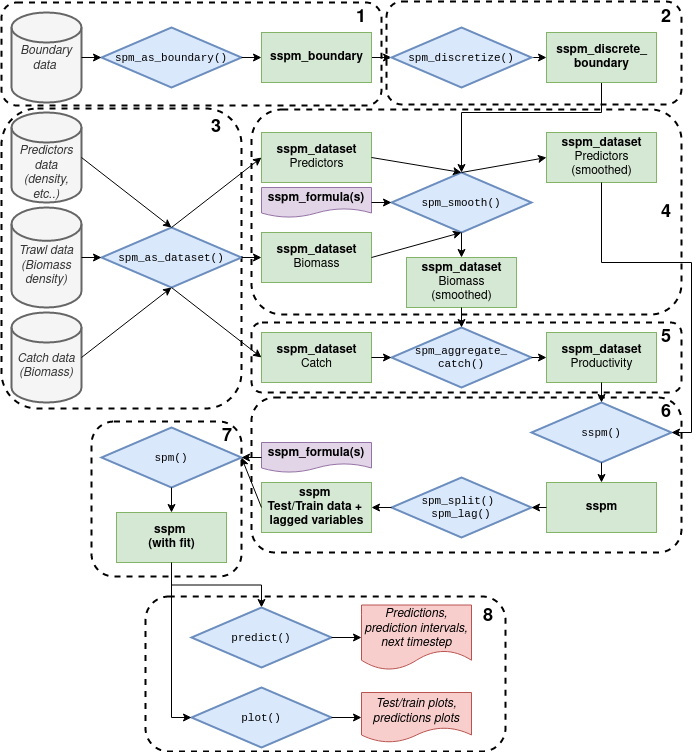The package follows an object oriented design, making use of the S4 class systems. The different classes in the package work together to produce a stepwise workflow.The sspm workflow.

1. The first pillar of the package’s design is the concept of boundary data, the spatial polygons that sets the boundary of the spatial model. The boundary data is ingested into a sspm_boundary object with a call to spm_as_boundary().

2. The boundary data is then discretized into a sspm_discrete_boundary object with the spm_discretize() function, dividing the boundary area into discrete patches.

3. The second pillar is the recognition of 3 types of data: trawl, predictors, and catch (i.e. harvest). The next step in the workflow is to ingest the data into sspm_dataset objects via a call to spm_as_dataset().

4. The first proper modelling step is to smooth the biomass and predictors data by combining a sspm_dataset, and a sspm_discrete_boundary. The user specifies a gam formula with custom smooth terms (see for more details). The output is still a sspm_dataset object with a smoothed_data slot which contains the smoothed predictions for all patches.

5. Then, catch is integrated into the biomass data by calling spm_aggregate_catch on the two sspm_dataset that contains catch and smoothed biomass. Productivity and (both log and non log) is calculated at this step.

6. The next step consists in combining all relevant datasets for the modelling of productivity (i.e. the newly created productivity dataset and the predictor(s) dataset(s)) with a call to sspm(). Additionally, the user may apply lags to the variables with spm_lag() and determine the split between testing and training data with spm_split().

7. The second modelling step consists in modelling productivity per se. Once again, a gam formula with custom syntax is used (see for more details).

8. The resulting object contains the model fit. Predictions can be obtained using the built-in predict() method, and plots with the plot() method.